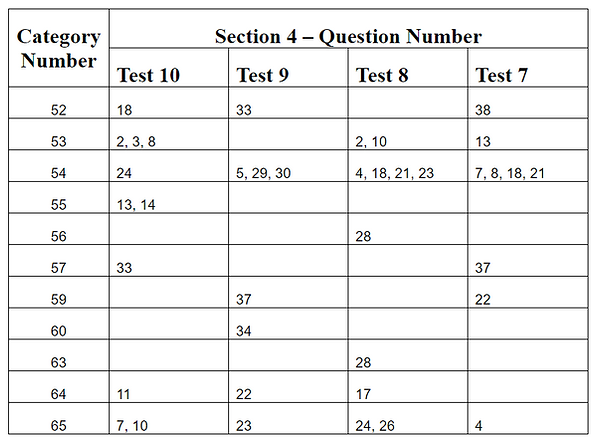Synopsis of Sections 3 and 11

## Section 3 - Word Problems on Linear Equations and Inequalities

There are several word problems on linear equations and linear inequalities on the SAT Math Section 3 and Section 4. The approach to solve these word problems is relatively straightforward. They are an easy win for a student who is familiar with the types of word problems and the steps to solve them.

Section 3 of this book is devoted to word problems on linear equations and linear inequalities with one or two variables, and word problems on system of linear equations and linear inequalities. The categories in this section contain examples and practice questions on various types of word problems that appear on the SAT. There are total of 72 practice questions in this section.

Below table shows all the related questions that appeared on SAT Math Tests 7 through 10 and their mapping to categories in Section 3 of this book.## Section 11 - Data Analysis and Interpretation

Every SAT Math Section 4 has several questions on topics related to probability, reading and interpreting graphs and events, statistical measures, and interpretation of data in studies and surveys. Section 11 of this book covers all the concepts that are required to answer the questions on the above mentioned topics. There are total of 131 practice questions in this section.

There may be questions on the SAT Math Section 4 that require analyzing multiple statistical measures in one data set or comparing multiple statistical measures in two data sets. This section contains a unique category, with examples and practice questions, that describes how to approach such questions.

Below table shows all the related questions that appeared on SAT Math Tests 7 through 10 and their mapping to categories in Section 11 of this book.